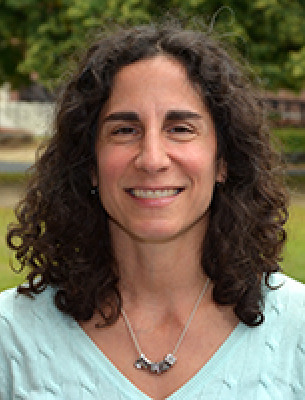# Mary Ann Barbato

PROFESSOR
Mathematics School of Health and Natural SciencesOffice: Edgerly Hall 301A
Office Hours
Fall 2023

Tuesday, Thursday, and Friday 12:30pm - 1:30pm

## Courses Taught

Basic Math I (MATH 0100)
Basic Math II (MATH 0200)
Introduction to Functions (MATH 1250)
Precalculus (MATH 1300)
Informal Number Theory (MATH 1500)
Informal Math Modeling (MATH 1600)
Discrete Math (MATH 1900)
Calculus I (MATH 2300)
Calculus II (MATH 2400)
Linear Algebra (MATH 2600)
Calculus III (MATH 3300)
Math Seminar (MATH 3900)
Abstract Algebra (MATH 4300)
PreK-12 Math Educators
Math Across the Curriculum
Graph Theory

## Background

Ph.D. in Mathematics, University of Rhode Island, 2001

Mathematics Education
Graph Theory

K1,3 – subdivision Tolerance Representations with Tolerance 1 and 2

Bulletin of the Institute of Combinatorics and Its Applications, Vol. 82, p. 21-29, Feb. 2018.  Co-author Nancy Eaton, University of Rhode Island Mathematics Department.

K1,3 – subdivision Tolerance Representations of Cycles

Bulletin of the Institute of Combinatorics and Its Applications, Vol. 65, p. 57-71, May 2012.  Co-author Nancy Eaton, University of Rhode Island Mathematics Department.

The Institute of Combinatorics and its Applications, ICA